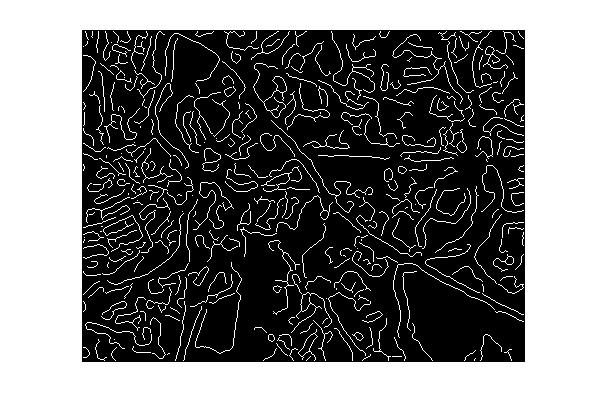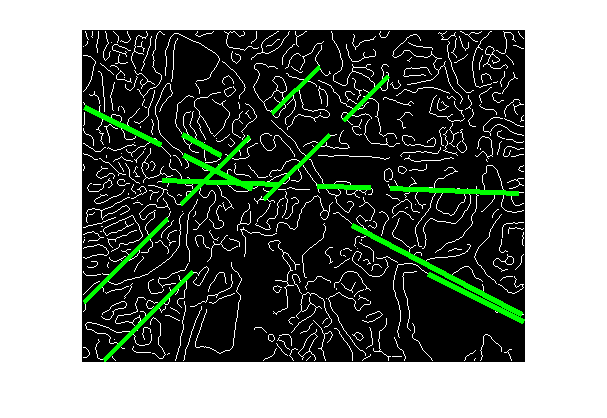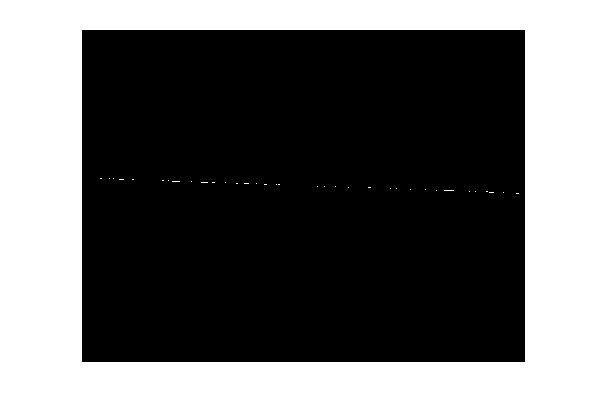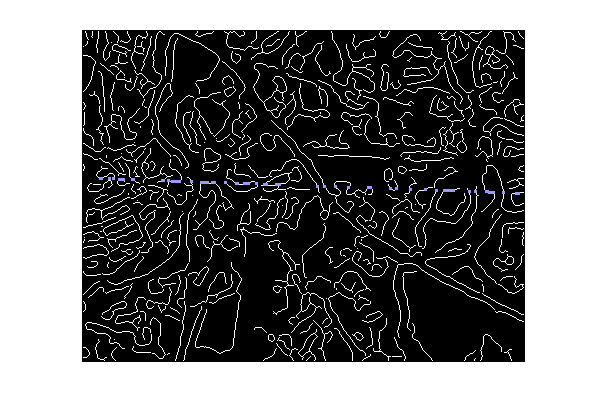# Showing image pixels associated with a Hough transform bin

Someone here recently puzzled over the output of the Hough transform functions in the Image Processing Toolbox. The Hough transform is used to detect line segments, typically in binary images. For the example we were looking at, some of the detected "line segments" didn't seem to correspond to line segments at all.

Let me show you what I mean:

I = imresize(imread('concordorthophoto.png'),0.15);
bw = edge(I, 'canny', [], 2);
imshow(bw)The function hough computes the Hough transform; houghpeaks finds peaks in the Hough transform; and houghlines detects line segments corresponding to the peaks.

[H,theta,rho] = hough(bw);
peaks  = houghpeaks(H,5);
lines = houghlines(bw,theta,rho,peaks);

Now superimpose the detected line segments on the original binary image.

imshow(bw)
hold on
for k = 1:numel(lines)
x1 = lines(k).point1(1);
y1 = lines(k).point1(2);
x2 = lines(k).point2(1);
y2 = lines(k).point2(2);
plot([x1 x2],[y1 y2],'Color','g','LineWidth', 4)
end
hold offSeveral of the green line segments shown above don't seem to correspond to any particular linear feature in the binary image. So why are they there?

Well, this is a fairly "busy" binary image, with many white pixels belonging to small, curvy features. These pixels do add up in the Hough transform accumulator bins. If you draw a line in any direction across this image, you'll find a lot pixels that lie on the line. All these pixels will contribute to the same accumulator bin.

I got to thinking that it would help to be able to visualize this in some way. That is, how can we see all the white pixels to contribute to a given accumulator bin?

Inside houghlines.m there's a subfunction called houghpixels that does this computation. I've pulled this out into an M-file called hough_bin_pixels.m. You give it a binary image, the theta and rho vectors returned by hough, and the row/column coordinates of a particular bin. It returns a new binary image containing only the white pixels that contributed to that particular bin.

For example, here are the white pixels corresponding to the fifth peak in the example above:

bw2 = hough_bin_pixels(bw, theta, rho, peaks(5,:));
imshow(bw2)Let's overlay those pixels in color on the original image. (imoverlay is a function available on the MATLAB Central File Exchange.)

rgb = imoverlay(bw, imdilate(bw2,ones(3,3)), [.6 .6 1]);
imshow(rgb)(I used dilation above to "thicken" the overlay pixels so that they are easier to see.)

So I think the Hough transform is not very useful for an image like this. It won't distinguish well between the linear features and the large number of other small features that also contribute to the accumulator bins.

Published with MATLAB® 7.2

|